# Python數據分析--Numpy常用函數介紹(2)CSV（Comma-Separated Value，逗號分隔值）格式是一種常見的文件格式。通常，資料庫的轉存文件就是CSV格式的，文件中的各個欄位對應於資料庫表中的列。

1、保存或創建新文件

```import numpy as np

i = np.eye(3) #eye(n)函數創建n維單位矩陣
print(i)
np.savetxt('test.txt', i) #savetxt()創建並保存test.txt文件```
`savetxt()函數，如果有已經文件則更新，如目錄中沒有，則創建並保存test.txt文件`

```[[1. 0. 0.]
[0. 1. 0.]
[0. 0. 1.]]```

1）先在保存程式的目錄下創建一個名稱為data.csv的文件，並設置數據如下圖：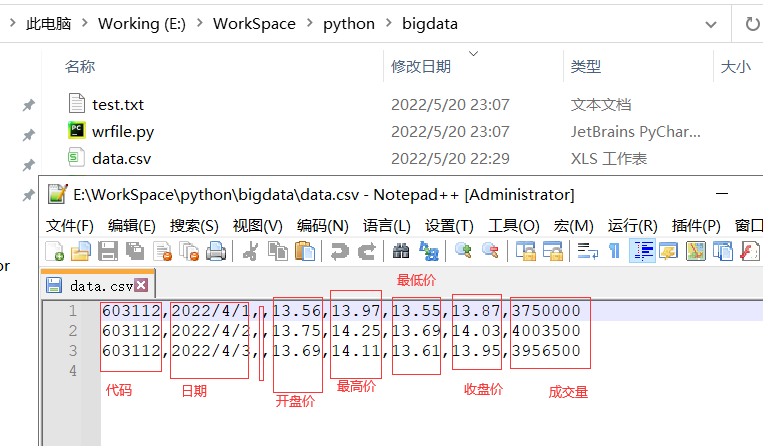2）讀取文件，如下：

`c,v=np.loadtxt('data.csv', delimiter=',', usecols=(6,7), unpack=True)`

usecols 的參數是一個元組，以獲取第7欄位至第8欄位的數據，也就是上述文件中 股票的收盤價和成交量數據。 unpack 參數設置為 True ，是分拆存儲不同列的數據，即分別將收盤價和成交量的數組賦值給變數c和v。

3、常見的函數

```import numpy as np

i = np.eye(3) #eye(n)函數創建n維單位矩陣
print(i)
np.savetxt('test.txt', i) #savetxt創建並保存test.txt文件

#讀取csv文件
"""usecols 的參數為一個元組，以獲取第7欄位至第8欄位的數據，也就是股票的收盤價和成交量數據。 unpack 參數設置為 True ，是分拆存儲不同列的數據，即分別將收

vwap = np.average(c, weights=v)  #調用了average函數,將v作為權重參數使用，
print(vwap)
print('\n')
print( np.mean(c)) #算術平均值
print('\n')
t = np.arange(len(c))
print( t )
print('\n')
twap =np.average(c, weights=t) #按時間權重
print( twap )
print('\n')
# 獲取第4欄位至第5欄位的數據，即股票的最高價和最低價

print ( np.max(h)) #獲取最大值max()
print ( np.min(l)) #獲取最小值min()
print('\n')
print( np.ptp(h) ) # 用ptp()函數計算了極差，即最大值和最小值之間的差值
print( np.ptp(l) )
print('\n')
print( np.median(c)) # 中位數median()函數，即多個數據中，處於中間的數
print( np.msort(c))#msort(( ))函數對價格數組進行排序,可以驗證上述中位數
#方差的計算
variance = np.var(c) #方差函數var()
print(variance)```

603112,2022-4-1,,13.56,13.97,13.55,13.87,3750000
603112,2022-4-2,,13.75,14.25,13.69,14.03,4003500
603112,2022-4-3,,13.69,14.11,13.61,13.95,3956500
603112,2022-4-4,,14.3,14.3,13.73,13.89,4250000
603112,2022-4-5,,14.1,14.5,13.93,14,4013500
603112,2022-4-6,,14.5,15.4,14.35,15.4,9056500
603112,2022-4-7,,16,16.94,15.85,16.94,3750000

4、股票的收益率等

```import numpy as np

#讀取csv文件

#股票的簡單收益率
# diff 函數可以返回一個由相鄰數組元素的差值構成的數組
results = np.diff(c)
print(results)
print('\n')
results1 = np.diff(c)/c[:-1]*100  #相對前一天的漲幅
print(results1)
print('\n')
Standard_deviation =np.std(results) # 計算出標準差
print(Standard_deviation)```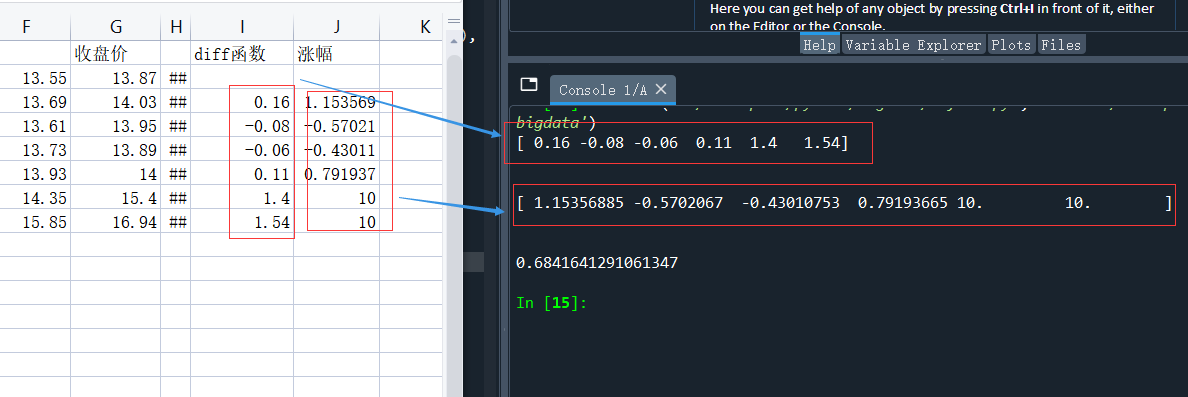5、對數收益與波動率

1）對數收益：log 函數得到每一個收盤價的對數，再對結果使用 diff 函數即可，

```logreturns = np.diff( np.log(c) )
print(logreturns)```

`[ 0.01146966 -0.00571839 -0.00431035  0.00788817  0.09531018  0.09531018]`

2) where的作用

where 函數可以根據指定的條件返回所有滿足條件的序列索引值，比如上述logreturns中有兩個小於0的數據。

```posretindices = np.where(results1 > 0)
print('Indices with positive returns1',posretindices)```

`Indices with positive returns1 (array([0, 3, 4, 5], dtype=int64),)`

3）波動率：波動率=對數收益率的標準差除以其均值，再除以交易周期倒數的平方根。下麵代碼分別為以年、月進行統計的波動率.

```annual_volatility =(np.std(logreturns)/np.mean(logreturns))/np.sqrt(1./252.)#使用浮點數才能得到正確的結果
print ( annual_volatility )
#月波動率
month_volatility =(np.std(logreturns)/np.mean(logreturns))/np.sqrt(1./12.)
print ( month_volatility )```

6、日期分析

`dates, c=np.loadtxt('data.csv', delimiter=',', usecols=(1,6), unpack=True) #讀取下標為1、6的數據，分別存入到dates和c數列中。`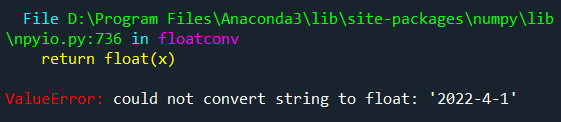```import numpy as np
from datetime import datetime

def datestr2num(s): #定義一個函數
return datetime.strptime(s.decode('ascii'),"%Y-%m-%d").date().weekday()
#decode('ascii') 將字元串s轉化為ascii碼

#讀取csv文件
print(dates)```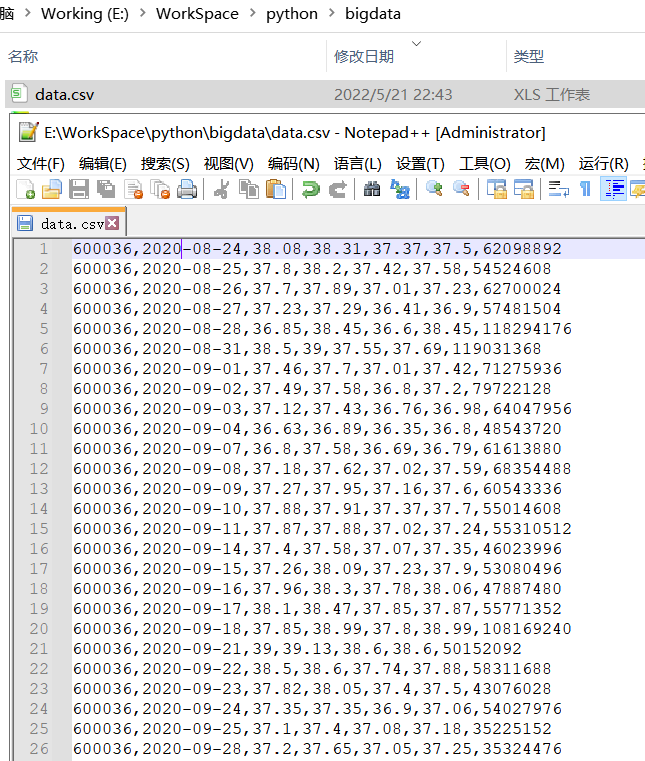（註意：較原來少了一列空格列）

```import numpy as np
from datetime import datetime

def datestr2num(s): #定義一個函數
return datetime.strptime(s.decode('ascii'),"%Y-%m-%d").date().weekday()
#decode('ascii') 將字元串s轉化為ascii碼

#讀取csv文件
converters={1:datestr2num},unpack=True)
print(dates)

print(len(dates)) #統計導出的天數``````averages = np.zeros(5) #創建包含5個元素的數組,保存交易日收盤價，0-4分別代表周一到周五五個交易日
for i in range(5):  #遍歷0到4的日期標識
indices =np.where(dates==i)   #where函數得到各工作日的索引值並存儲在 indices 數組
prices=np.take(c,indices)   #take函數獲取各個工作日的收盤價。
avg= np.mean(prices) #每個工作日計算出平均值存放在 averages 數組
averages[i] = avg  #每個工作日計算出平均值存放在 averages 數組
print('day', i)
#print('prices', prices)
print("Average", avg)

print(averages)```

```import numpy as np
from datetime import datetime

def datestr2num(s): #定義一個函數
return datetime.strptime(s.decode('ascii'),"%Y-%m-%d").date().weekday()
#decode('ascii') 將字元串s轉化為ascii碼

#讀取csv文件
converters={1:datestr2num},unpack=True)

averages = np.zeros(5) #創建包含5個元素的數組,保存交易日收盤價，0-4分別代表周一到周五五個交易日
for i in range(5):  #遍歷0到4的日期標識
indices =np.where(dates==i)   #where函數得到各工作日的索引值並存儲在 indices 數組
prices=np.take(c,indices)   #take函數獲取各個工作日的收盤價。
avg= np.mean(prices)
averages[i] = avg  #每個工作日計算出平均值存放在 averages 數組,共有5個數值
print('day', i)
#print('prices', prices)
print("Average", avg)

print(averages)
print('\n')

print('the top close price:',np.max(c)) #最高收盤價
print('the low close price:',np.min(c)) #最低收盤價
print('\n')

top = np.max(averages)  #找出averages數列中的最大值
print ("Highest average", top)
print ("Top day of the week", np.argmax(averages)) #argmax函數返回的是averages數組中最大元素的索引值
print('\n')

bottom = np.min(averages) #找出averages數列中的最小值
print ("Lowest average", bottom)
print ( "Bottom day of the week", np.argmin(averages))#argmin函數返回的是averages數組中最小元素的索引值```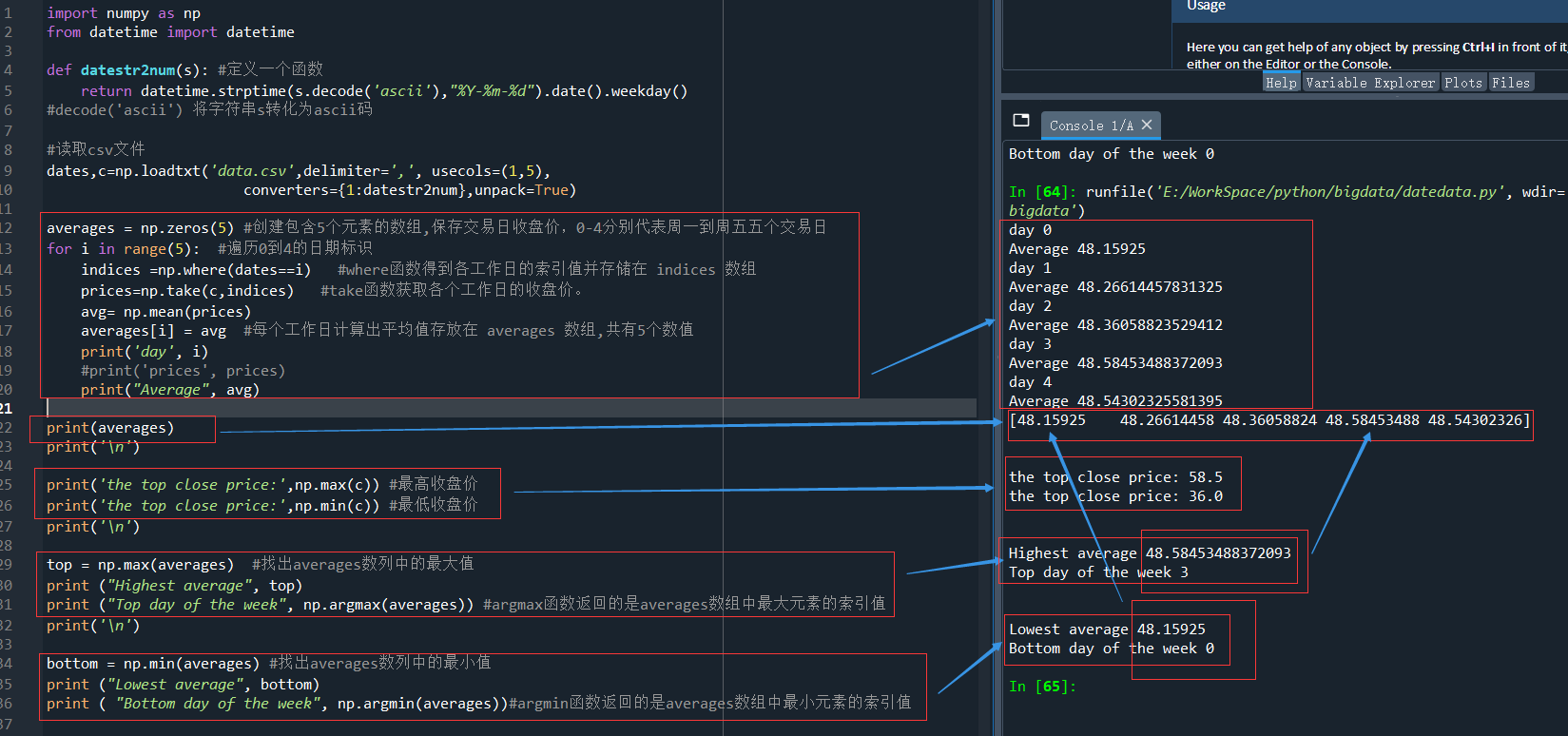本篇初步導入了真實的股票交易信息，並利用numpy常見函數對進行了初步的計算，列舉了下列常用函數：

savetxt()創建並保存test.txt文件
np.average(c, weights=v) 加權平均,將v作為權重參數使用，
np.mean(c)) #算術平均值
np.max(h)) #獲取最大值max()
np.min(l)) #獲取最小值min()
np.ptp(h) ) 用ptp()函數計算了極值差，
np.median(c)) 中位數median()函數，即多個數據中，處於中間的數
np.msort(c))函數對價格數組進行排序,
np.var(c) 方差函數var()
np.diff(c) 函數可以返回一個由相鄰數組元素的差值構成的數組
np.std(results) # 標準差
np.diff( np.log(c) )
np.where(results1 > 0) 選擇
np.sqrt()#平方根sqrt(),浮點數
s.decode('ascii') 將字元串s轉化為ascii碼
np.take(c,indices) #take函數獲取各個工作日的收盤價。
np.argmax(averages)) #argmax函數返回數組中最大元素的索引值
np.argmin(averages))#argmin函數返回數組中最小元素的索引值• 出現mysqld: Can't create directory 'D:\Environment\mysql-5.7.37 \data' (Errcode: 2 - No such file or directory)極大原因是my.ini的配置有問題 my.ini配置 [mysqld] based ...
• 分享嘉賓：牟娜 阿裡巴巴 高級演算法工程師 編輯整理：孫鍇 內容來源：DataFun AI Talk《定向廣告新一代點擊率預估主模型——深度興趣演化網路》 出品社區：DataFun 導讀： 本次帶給大家分享是阿裡媽媽在2018年做的模型上的創新——深度興趣演化網路（Deep Interest Evol ...
• B+樹索引的正確使用 索引並不是越多越好，索引創建越多，MySQL維護的代價越高，如果SQL未能完全使用到索引，創建索引的意義是不大的。 適用條件 表x，創建索引a,b,c。主鍵y。 全值匹配 select * from x where a = '' and b = '' and c = '' 當我 ...
• 導讀： 線上社交媒體平臺的發展，帶來了細粒度檢索、視頻語義摘要等媒體智能服務的巨大需求。現有的視頻理解技術缺乏深入的語義線索，結合視頻中人物的社交關係才能更完整、準確地理解劇情，從而提升用戶體驗，支撐智能應用。這裡主要介紹我們將動態分析和圖機器學習相結合，圍繞視頻中的人物社交關係網路所開展的兩個最新 ...
• 問題描述：發現主庫操作數據從庫沒有變動問題，可能原因是從庫重啟導致的無法同步問題。 排查思路： 1、查看主從複製狀態 發現從庫的IO和SQL進程都是no(正常狀態應該是yes) 註意：mysql replication中slave機器上有兩個關鍵進程，死一個都不行，一個是slave_sql_runn ...
• Toolbar 新建項目會預設顯示ActionBar，ActionBar根據項目指定的主題來顯示，android:theam指定主題，在res/values/theams.xml中定義主題，因為用toolbar代替ActionBar，所以將parent主題設置為不帶ActionBar的主題，如下： ...
• 在瀏覽器測試JavaScript的方法 JSFiddle Cross Browser Testing Tool Karam + Jasmine + Google Chrome CodePen JSBin Liveweave 測試JavaScript是一件很痛苦的事情。很多工具、技術和框架已經被開發出 ...